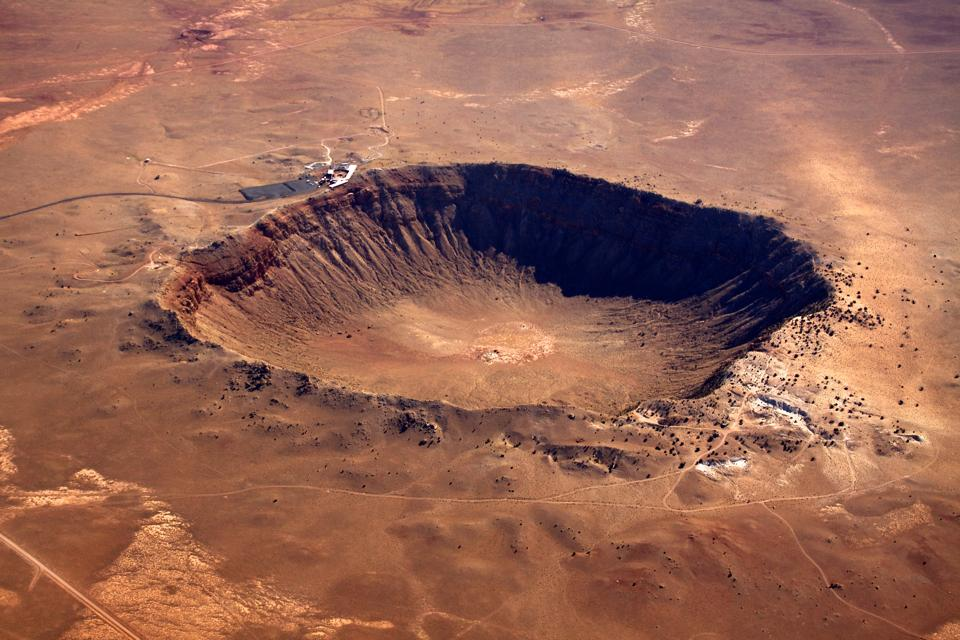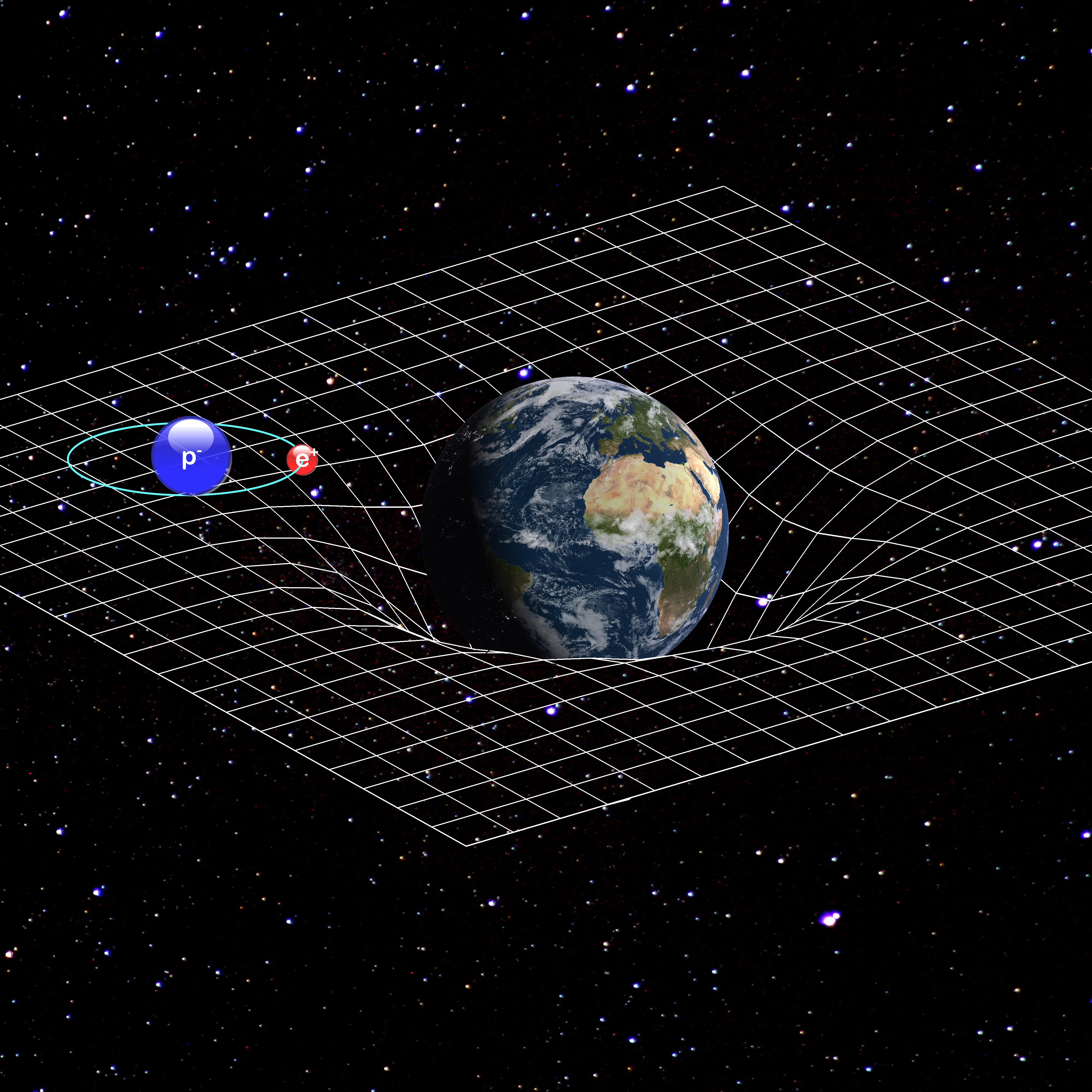Skip to main content

## Akoranga 4 Mahi Tuatahi

1. Open Quizizz on your device (phone or laptop)
2. Get ready to play!

https://quizizz.com/admin/quiz/5c110c53e13e0f001a388fbb/meteors-comets-and-asteroids

## Ngā Whāinga Ako

1. Recall key terminology around meteorites
2. Describe factors that affect the size and shape of an impact crater

Write the date and ngā whāinga ako in your book

## Pātai

• Do you recall the Law of Conservation of Energy? Check with the person next to you!

### Whakatika

Energy cannot be created or destroyed, it can only be transferred or transformed.## Pātai: Describe These Transformations

1. Miles is playing football and kicks the ball into the air upfield to a striker.
2. Toby gets up in the morning to go for a run. He eats three Weet-Bix before he goes.
3. Phoenix goes bungee jumping near Wanaka.

Write the answers in paris, in your book.

### Whakatika

1. Kinetic (foot) –> elastic + sound + heat –> kinetic + gravitational (ball) –> kinetic + sound + heat + pontential as the striker catches the ball.
2. Chemical –> potential + kinetic as he gets up –> kinetic as he runs
3. Potential –> kinetic –> elastic potential at the bottom

## Pātai: Energy in Meteors

What energy transformations do meteoroids undergo as they fall towards and onto a planet/moon?### Whakatika• In space they have gravitational potential & kinetic energy
• As they fall, gravitational potential energy is transformed into more kinetic energy
• Some of this kinetic energy is dissipated as heat/light/sound as it impacts the atmosphere

## Pātai

What happens to the rest of the kinetic energy if the meteor doesn’t completely break up in the atmosphere?### WhakatikaThe kinetic energy is transferred into the ground, creating an impact crater and throwing out debris!

## Gravitational Potential Energy / Pūngao tō ā-papa

The potential an object has to fall in a gravitational field.

\begin{aligned} E_{p} &= mass \times gravity \times height \
E_{p} &= m \times g \times h \end{aligned}

Pātai: What can we change about a meteoroid to give it more gravitational potential energy?

### Whakatika

1. Increase its mass
2. Increase its height (distance away from Earth) in the gravitational field## Kinetic Energy / Pūngao Neke

Energy an object in motion has

\begin{aligned} Energy &= \frac{1}{2} mass \times velocity^{2} \
E &= \frac{1}{2}m \times v^{2} \end{aligned}

Pātai: What can we change about a meteorite to give it more kinetic energy?

### Whakatika

1. Increase its mass!

\begin{aligned} E = \frac{1}{2} \times 10 \times 10^{2} = 500J \
E = \frac{1}{2} \times 20 \times 10^{2} = 1,000J \end{aligned}

1. Increase its velocity (has a greater effect)!

\begin{aligned} E = \frac{1}{2} \times 10 \times 10^{2} = 500J \
E = \frac{1}{2} \times 10 \times 15^{2} = 1,125J \end{aligned}

## Akoranga 5 Mahi Tuatahi

1. Brainstorm some characteristics of a crater that could change depending on the energy of the impact?

### Whakatika

• Crater depth
• Crater width
• Amount/volume of debris ejected
• Distance that debris is ejected

## Conservation of Energy

Typically to increase the energy of an impact in a simulation we increase the height that it is dropped from, thereby giving it more gravitational potential energy to be converted to kinetic energy (increasing its impact velocity).

\begin{aligned} E_{p} &= E_{k} \
m \times g \times h &= \frac{1}{2}m \times v^{2} && \text{Mass cancels out} \
g \times h &= \frac{1}{2} \times v^{2} && \text{Re-arrange for v} \
2 \times g \times h &= v^{2} \
\sqrt{2 \times g \times h} &= v \end{aligned}

### Theoretical vs Real-World

\begin{aligned} v = \sqrt{2 \times g \times h} \end{aligned}• This gives the theoretical impact velocity of a rock dropped within Earth’s gravitational field.
• Pātai: What assumption are we making here?
• Whakatika: That energy is conserved
• Pātai: What happens to some of that energy?
• Whakatika: That some energy is lost to the atmosphere as light/heat/sound.
• Whakakapi/Conclusion: Real-world velocity is less than the theoretical maximum velocity.

## Pātai

\begin{aligned} v &= \sqrt{2 \times g \times h} \
E_{k} &= \frac{1}{2} \times m \times v^{2} \end{aligned}

1. Calculate the impact speed of an object dropped from $1m$
2. Calculate the impact speed of an object dropped from $2m$
3. Calculate the impact speed of an object dropped from $5m$
4. Calculate the impact kinetic energy of a $10kg$ object travelling at $3000ms^{-1}$
5. Calculate the impact kinetic energy of a $750kg$ object travelling at $5000ms^{-1}$

## Ngohe: Google Earth Tour1. Open Google Classroom and find the Google Earth link
2. Follow through the tour – make sure to read more on each stop!

## Ngohe: Quizizz

https://quizizz.com/admin/presentation/5fcd762e8e241c001b80de03/meteoroids-meteor-or-meteorites